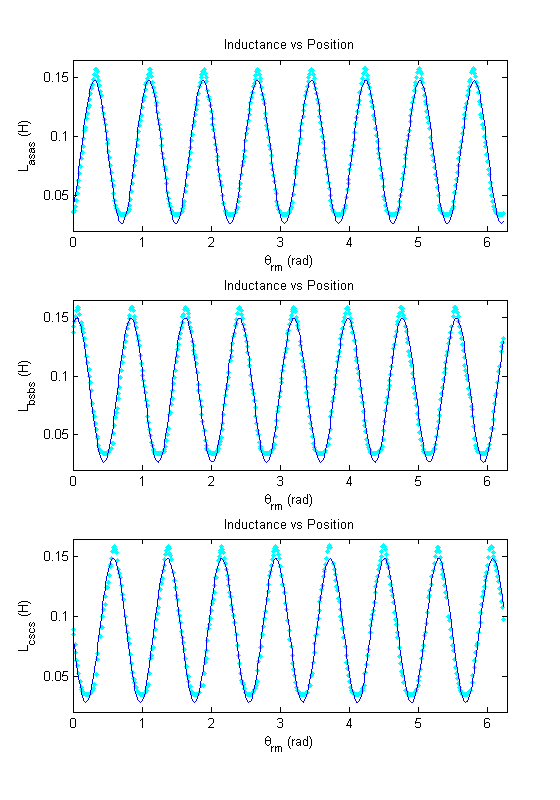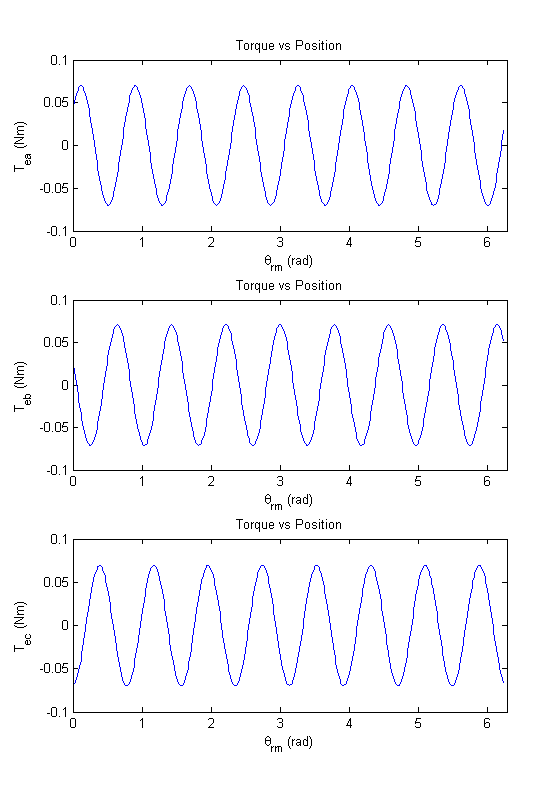# Lab 4a Tips: Variable Reluctance Stepper Motor

## In-lab

• RT = 8
• r ≈ 20 Ω
• Sample plot of inductance vs. position.## Post-lab

• The fit8.m file is provided for you in the lab4a folder. As long as this function is in your Matlab working directory you can call it from your post-lab m-file with `fit8(las,qrma);`. If you didn't save a copy of fit8.m to your account during lab you can make your own from the code at the end of Appendix 2.
• A short note on how to derive torque equation can be downloaded from [PDF]. This will be useful for completing last part of the Post-lab.
• Use the 'subplot' and 'orient tall' commands to generate your plots. The 'subplot' command lets you place multiple axes on one figure. The command 'orient tall' expands the plot vertically to fill the page when you print.

``` subplot(3,1,1) %plot las or Tea vs qrma subplot(3,1,2) %plot lbs or Teb vs qrmb subplot(3,1,3) %plot lcs or Tec vs qrmc orient tall ```